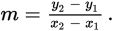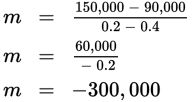# SAT Math Multiple Choice Question 399: Answer and Explanation

### Test Information

Question: 399

 Price per Pencil Projected Number of Units Sold \$0.20 150,000 \$0.25 135,000 \$0.30 120,000 \$0.35 105,000 \$0.40 90,000 \$0.45 75,000

9. Generally, the price of an item is a good indicator of how many units of that item will be sold. The lower the price, the more units will be sold. A marketing department develops a table showing various price points and the projected number of units sold at that price point. Which of the following represents the linear relationship shown in the table, where x is the price and y is the number of units sold?

• A. y = 0.03x + 150,000
• B. y = 300,000x + 75,000
• C. y = -300,000x + 90,000
• D. y = -300,000x + 210,000

Explanation:

D

Difficulty: Medium

Category: Heart of Algebra / Linear Equations

Strategic Advice: Take a quick peek at the answer choices. The equations are given in slope-intercept form, so start by finding the slope.

Getting to the Answer: Find the slope by substituting two pairs of values from the table (try to pick easy ones, if possible) into the slope formula,Keep in mind that the projected number of units sold depends on the price, so the price is the independent variable (x) and the projected number is the dependent variable (y). Using the points (0.2, 150,000) and (0.4, 90,000), the slope isThis means you can eliminate A and B because the slope is not correct. Don't let B fool you—the projected number of units sold goes down as the price goes up, so there is an inverse relationship, which means the slope must be negative. To choose between C and (D), you could find the y-intercept of the line, but this is a fairly time-intensive process. Instead, choose any pair of values from the table, such as (0.2, 150,000), and substitute into C and (D) only. Choice (D) is correct because 150,000 = -300,000(0.2) + 210,000 is a true statement.